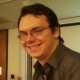## Koszul Duality and Lie Algebroids

Something has been bothering me about Koszul duality lately.  Well, technically many things have been bothering me about it, but here’s a particular thing that has been bothering me.   Usual (homological) Koszul duality assigns to an augmented$R$-dga$A$ the algebra$A^!:=RHom_A(R,R)$.  Since$R$ is central,$A^!$ is again an augmented$R$-dga, and so$A^{!!}$ makes sense.  In many (all?) cases,$A^{!!}\sim A$ (hence, duality).

I am interested in the case when$R$ is no longer central in$A$.  Then an action of$A$ on$R$ no longer is the same as an augmentation map, so we only assume we have a left$A$-module structure on$R$ (where the contained copy of$R$ acts by mutliplication).  We can still form$A^!$ in the same way; however,$A^!$ no longer contains$R$, so it seems impossible to form$A^{!!}$.

However, I have reason to suspect that one should be able to form$A^{!!}$, and that it should often be quasi-isomorphic to$A$.  My evidence in this direction is a paper of Positsel’skii’s, “Nonhomogeneous Quadratic Duality and Curvature”, wherein he shows that for a very narrow class of algebras$A$ which both contain and act on$R$, that$A$ can be recovered from$A^!$.

An important case of this duality is for the ring of differential operators$\mathcal{D}$ on a smooth curve.  This has a canonical left action on$\mathcal{O}$, and so$\mathcal{D}^!:=RHom_\mathcal{D}(\mathcal{O},\mathcal{O})$ exists, and is in fact the deRham complex.  So, a specific case of my question is: is there a sense in which the deRham complex$\Omega$ of a space acts on$\mathcal{O}$, such that something like$RHom_\Omega(\mathcal{O},\mathcal{O})\sim \mathcal{D}$?

More generally, one can replace$\mathcal{D}$ with the universal enveloping algebra$\mathcal{U}$ of any Lie algebroid over$\mathcal{O}$$\mathcal{U}^!$ can still be defined, and is the complex which computes the Lie algebroid cohomology.

Both of these examples do fall under Positsel’skii’s explicit duality.  The problem is that the framework of this duality isn’t self-contained.  It takes filtered algebras which aren’t too far from being quadratic algebras, and sends them to commutative dgas whose underlying graded algebra is quadratic.  Then there is a different construction which takes quadratic commutative dgas to filtered algebras which are almost quadratic, and these two functors induce an equivalence of categories.

What I want, though, is for these two dualities to be facets of the same duality.  Maybe its not possible, but I imagine that people have thought about this, at least in the specific case of Lie algebroids.

### 4 Responses to “Koszul Duality and Lie Algebroids”

1.Scott Carnahan Says:

Bruno Vallette has some papers on general manifestations of Koszul duality, which in your case would take the associative algebra D to the coassociative coalgebra of exterior powers of the tangent sheaf (grain of salt – I haven’t done any calculations). Unfortunately, there is a nontrivial amount of translation to be done between your case and the properad/coproperad language in the papers.

2.David Ben-Zvi Says:

Hi — the Koszul duality for D-modules is explained nicely
in the article of Kapranov “On DG modules over the de Rham complex..”
Indeed O is a de Rham module corresponding to D —
it might be easier to see by writing down the full
de Rham complex of D as a left D-module, and checking it
is quasiisomorphic to O.

The way he treats the duality also generalizes to Lie algebroids:
it makes life much easier to not start with D and de Rham,
or U(L) and Chevalley(L), but to look at the Rees algebra of D which
is now a graded algebra, and its Koszul dual which is the exterior
algebra of L^* adjoined the Chevalley differential as an
extra variable. One gets the duality you want by localizing
wrt the extra variable on both sides.

As for the general theory I think Quillen’s article
on rational homotopy theory is really inspiring –
he works out the commutative/Lie Koszul duality, but
without calling it such. The Lie algebroid/Chevalley
case is a relative version of this. Quillen’s
article also gives context as to why we’re taking bar/cobar
complexes in Koszul duality — it corresponds to looping
and delooping spaces (the Lie algebra side can be thought of
as the Lie algebra of a loop space – which is a group roughly speaking — and the commutative algebra side corresponds to the original space,
which is the delooping or classifying space of its loop space.)
In geometry this corresponds to the modern approach to deformation
theory – starting from a commutative algebra you calculate
a (dg) Lie algebra, which is its tangent space (complex),
while from a Lie algebra you calculate the classifying space
of its formal group, a.k.a. the space of solutions to the Maurer-Cartan equation.

3.Greg Muller Says:

Thanks for the answers! While I don’t have any comments until I get a chance to look at some of these papers (printing is busted here again), heres another related question. What is the significance of a dga being isomorphic to its Koszul dual?

Given a smooth manifold$M$, one can talk about$\mathcal{D}(\Omega(M))$, the ring of differential operators on the dga of differential forms. Equipping$M$ with a riemannian structure determines a Hodge star, and conjugation with the Hodge star is an involution of$\mathcal{D}(\Omega(M))$.

I am very curious about the fixed set of this involution, the differential operators which commute with the Hodge star. They came up when I was trying to find a universal property for the non-commutative Weil algebra (in the sense of Alexeev and Meinrenken). Given an invariant metric on G, and a metric-preserving action of G on a riemannian manifold M, I believe that the non-commutative Weil algebra maps into the sub algebra of$\mathcal{D}(\Omega(M))$ which commutes with the Hodge star.

Since the above Koszul duality swaps$\Omega(M)$ and$\mathcal{D}(M)$, I was hoping that it would map the self-dual differential operators to themselves, and maybe say something about why this algebra carries any topological significance.

4.sunacigu Says:

and are very important these observed prof dr mircea orasanu and ptof drd horia orasanu